# Texas Go Math Grade 4 Unit 2 Answer Key Number and Operations: Whole Number and Decimal Operations

Refer to our Texas Go Math Grade 4 Answer Key Pdf to score good marks in the exams. Test yourself by practicing the problems from Texas Go Math Grade 4 Unit 2 Answer Key Number and Operations: Whole Number and Decimal Operations.

## Texas Go Math Grade 4 Unit 2 Answer Key Number and Operations: Whole Number and Decimal Operations

Show What You Know

Check your understanding of important skills.

3-Digit Subtraction Within 1,000 Find the difference.

Question 1.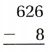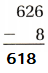Explanation:
Three digit subtraction
The difference of 626 and 8 is 618

Question 2.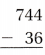Explanation:
Three digit subtraction
The difference of 744 and 36 is 710

Question 3.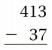Explanation:
Three digit subtraction
The difference of 413 and 37 is 376

Question 4.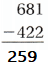Explanation:
Three digit subtraction
The difference of 681 and 422 is 259

Question 5.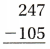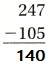Explanation:
Three digit subtraction
The difference of 247 and 105 is 140

Question 6.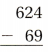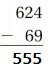Explanation:
Three digit subtraction
The difference of 624 and 69 is 555

Question 7.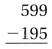Explanation:
Three digit subtraction
The difference of 599 and 195 is 404

Question 8.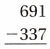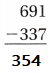Explanation:
Three digit subtraction
The difference of 691 and 337 is 354

Practice Multiplication Facts Find the product.

Question 9.
8 × 7 = ___________
7 × 8 = ___________
8 × 7 = 56
7 × 8 = 56
Explanation:
The product of both the equations are same

Question 10.
4 × 6 = ___________
6 × 4 = ___________
4 × 6 = 24
6 × 4 = 24
Explanation:
The product of both the equations are same

Question 11.
3 × 5 = ___________
5 × 3 = ___________
3 × 5 = 15
5 × 3 = 15
Explanation:
The product of both the equations are same

Question 12.
6 × 9 = ___________
9 × 6 = ___________
6 × 9 = 54
9 × 6 = 54
Explanation:
The product of both the equations are same

Model Division with Arrays Draw to complete each array. Then complete the number sentence.

Question 13.8 ÷ 4 = ___________Explanation:
2 x 4 = 8
Division and multiplication are inverse operations

Question 14.21 ÷ 3 = ___________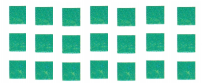Explanation:
3 x 7 = 21
Division and multiplication are inverse operations

Vocabulary Builder

Visualize It

Review Words:

• divide
• dividend
• division
• divisor
• factor
• multiple
• multiplication
• product
• quotient

Preview Words:

• compatible numbers
• Distributive Property
• partial product
• partial quotient
• remainder

Sort the words into the Venn diagram.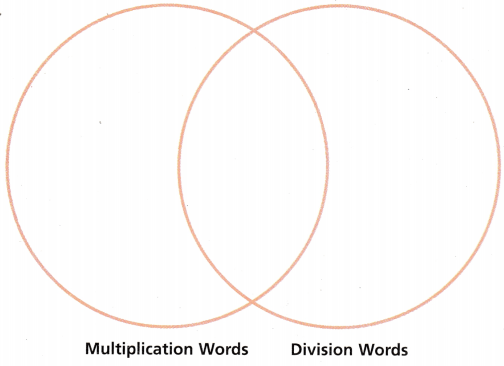Explanation:
The words are separated in the Venn diagram
according to the multiplication words and the division words

Understand Vocabulary

Write the word that answers the riddle.

Question 1.
I am the method of dividing in which multiples of the divisor are subtracted from the dividend and then the quotients are added together. _________________

Question 2.
I am the number that is to be divided in a division problem. _________________

Question 3.
I am the amount left over when a number cannot be divided equally. _________________

Question 4.
I am the method of multiplying in which the ones, tens, hundreds, and so on are multiplied separately and then the products are added together. _________________

Name _________________

Vocabulary
Sometimes when you multiply greater numbers, you can estimate the product by rounding factors to the greatest place value.
A movie theater is expanding its 218 seats to three times that number. About how many seats will the theater have?
Answer: nearest number of 218  is 200
200 x 3 = 600

Fill in the chart to estimate the product of 3 × 218.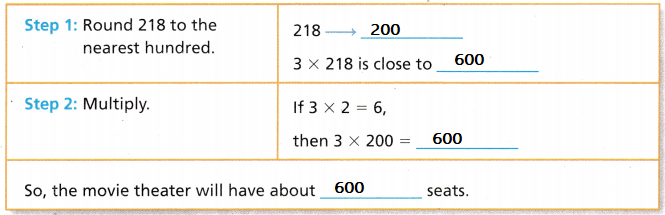nearest number of 218  is 200
200 x 3 = 600

Fill in the chart to estimate the product of 24 × 49.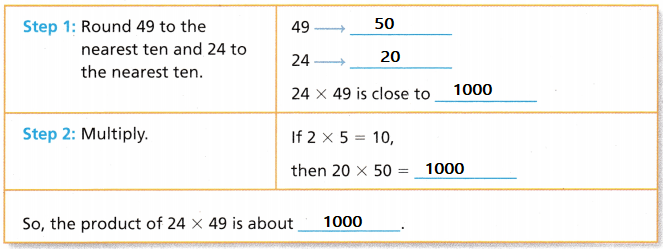Explanation:
Rounded the 24 to 20 and 49 to 50

Writing Write down a 1-digit and a 4-digit number and estimate the product.
Explanation:
8 is rounded to 10
2222 is rounded to 2000
10 x 2000 = 20000

Reading Look for this book in your library. Great Estimations, by Bruce Goldstone

Digit Division

Object of the Game Create a division problem with the smallest remainder.

Materials:

• 3 sets of 1-9 number cards
• One number cube labeled with digits 2, 3, 4, 5, 6, and 9

Set up:
Shuffle the number cards. Label a number cube with the digits 2, 3, 4, 5, 6, and 9.
Number of players 2

How to play

Question 1.
Player 1 picks three number cards. Player 2 tosses the number cube. Each player makes a dividend from the digits on the number cards. Players divide by the number on the number cube.Explanation:
6 divided by 2 is 3

Question 2.
Players earn points equal to the remainder. If the remainder is 0, the player earns no points.